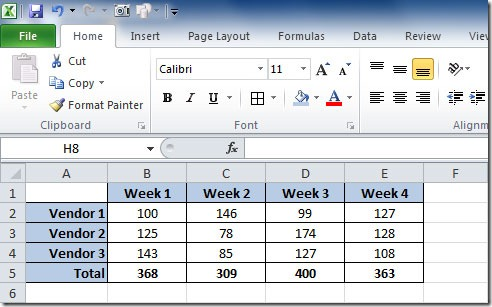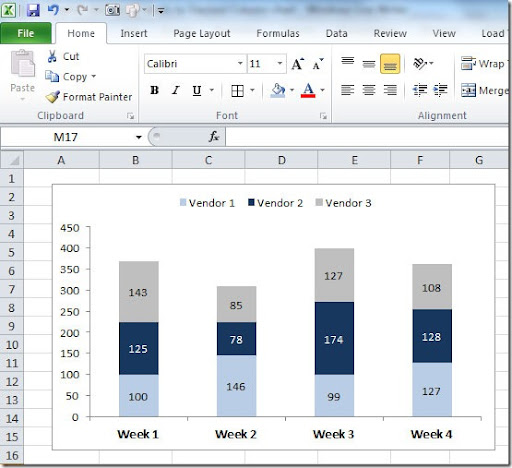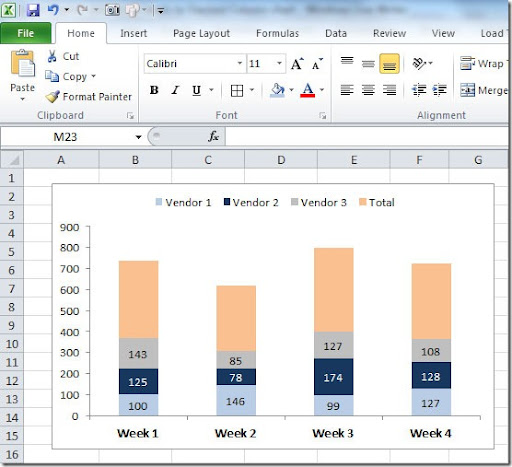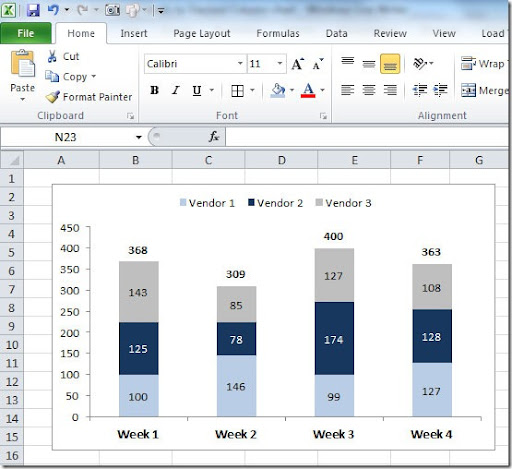### Convert numbers to minutes

If you have a series of values like this:

12.5
9.3
10.7

and want to convert this numbers to a minutes/seconds format you can use this formula:

=A1/(24*60)

This assumes that the values below are on cells A1:A3 and this formula is placed on cell B1 and copied down. You need to format the cells B1:B3 as Custom mm:ss to see this:

12:30
09:18
10:42

### Multiply range of values by a value

One of the most common tasks that we need to do in Excel is multiply a range of value on a sheet by a specific value, say, multiply a range of values by 100. This can be done very easily by following this steps. Say you have this sheet:In cell A5 you should put the value that you want to use to multiply the values on cells A1:D3. Now on cell A5 make a Ctrl+C (Copy) and select the cells A1:D3. Right-click on top of the range and select Paste Special, on Paste options select the Values and on Operation select Multiply. Click OK. Now your sheet should look like this:If instead of multipying the values by 100, you Add, Substract or Divide the values by the value that you select on another cell (in this case I used the cell A5).

### Get row number from a match

Excel has a function to locate a value on a range of cells and return the number of the row where the value is found, it’s the MATCH() function. The syntax is like this:

MATCH(lookup_value,lookup_array,match_type)

lookup_value is the value that we want to look for and lookup_array is the range of cells where we want to search. match_type can have 3 values:
1 - finds the largest value that is less than or equal to lookup_value. Lookup_array must be placed in ascending order;
0 - finds the first value that is exactly equal to lookup_value
-1 - finds the smallest value that is greater than or equal to lookup_value. Lookup_array must be placed in descending order.
If match_type is omitted, it will assume to be 1.
Here’s an example. We have the following table:We want to find the name Mary on the range of A2:A6. So we should use this formula:

=MATCH("Mary",A2:A6,0)

This will return 2 because the name Mary can be found on cell A2.

### Excel Charts - Add totals labels to Stacked Column chart

Let's say that I have the following table of data:I can make a Stacked Column chart with 3 series, one for each Vendor. The chart will look like this:But I want to display on the top of each bar, the total for the Week (the sum of each Vendor). To do that, I need to add a 4th series to the chart. For that I select the chart and right-click on top of it and select Source Data. On the Series tab, on the Series, click on the Add button to add a new series. For the Values range, select the cells where the values of Total are on our table. For the Name, select the cell where we have "Total". Click OK and you will get a chart like this:Select this new series on the chart, right click and choose Format Data Series. On the Data Labels tab, Label Contains mark Value. Then on the Patterns tab put Border-None and Area-None. Click OK. Now select the labels, right click on them, choose Format Data Labels and on the Alignment tab, Label Position choose Inside Base. Click OK. You need to adjust the scale of your chart so that it looks like the original one. In this case, I changed Maximum value to 450 instead of Automatic. As you can see, the “Total” series name still shows up on the Legend. You need to select the Total legend and delete. The final result will be this something like this:Hope this helps you create better charts in Excel!

### VBA – Define dynamic ranges

In VBA code we can define a dynamic range by checking the last row that has data on it. this can be defined in various ways. Here is a simple way of doing it:

Dim rng As Range
rng = Range("A12:C" & Worksheets("Sheet1").Range("C65535").End(xlUp).Row + 1)

This will get us the range A12:C200 if C200 is the last used cell in column C.
You can also define a range that gets all of the columns in row 1 that are used as column headers, like this:

Dim ws As Worksheet
Dim rng As RangeSet
ws = ActiveSheetSet
rng = Intersect(ws.Rows(1), ws.UsedRange)

This will get us the range of A1:D4 if columns A, B, C and D have data on it.

### VBA – Get font color Function

When we have colors on our sheets data and want, for instance, to count how many “red” words we have on our sheet, that is not possible because there is no formula in Excel to check for font colors. Instead we can create our own VBA Function to get the font color. It’s a very simple code. You have to insert it on a VBA module on your sheet.

Function GetFontColor(ByVal Target As Range) As Object
GetFontColor = Target.Font.ColorIndex
End Function

Then you can use it on your sheet like this:

GETFONTCOLOR(A2)

Below is an example on how you can use this function. In column C we put the font color of text in column A.To count the number of “red” words in column A we can simple to this:

COUNTIF(C2:C9,3)

“3” in the formula refers to the color red.